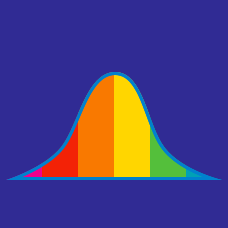Probability

# Data - Mode

$\{ 13, 7, 13, 7 \}$

What is the mode of the above set?

Find the mode for the following list of values: $8, 12, 25, 8, 8, 12, 25, 25, 8.$

9 students took a test that was scored out of 10.
2 of them got a score of 0,
3 of them got a score of 4,
and 4 of them got a score of 8.

What is the mode score?

$\{ 9, 1, 12, 15, 17, 9, 5, 1, 9, 10 \}$

The above is a list of the number of problems that Benny worked on in the past 10 days. What is the mode?

$\{ 3, 7, 10, 19, 19 \}$

What is the mode of the above set?

×